# RFEM – Online Manual

Online manuals, introductory examples, tutorials, and other documentation.

# 7.2.2.1 FE Mesh Settings

#### FE Mesh Settings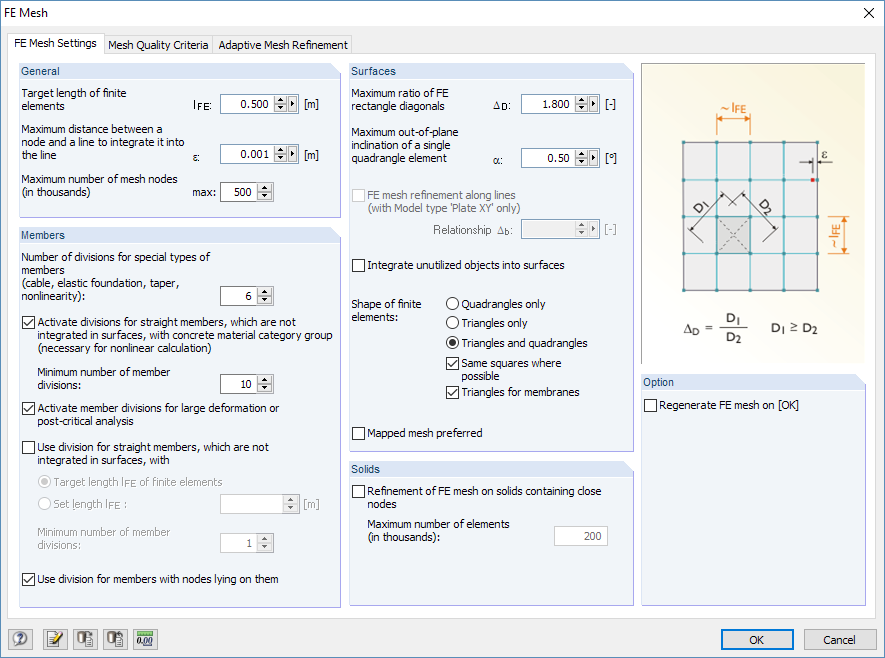Figure 7.10 FE Mesh dialog box, FE Mesh Settings tab
General

The Target length of finite elements controls the global target length of the mesh. The finer the mesh is, the more precise the results generally are. However, the amount of data to be calculated and the computing time are increased as well, since additional equations must be solved for any additional FE node. Furthermore, singularity effects occur more frequently in a fine-meshed FE mesh.

The discretization is important for the subsequent FE analysis: A mesh size that is too fine slows down the calculation without significantly improving the quality of results. A target length that is too long cannot determine boundary conditions in a satisfying way. The following recommendation can be made for the appropriate lateral length of finite elements: Eight to ten finite elements should be generated between the boundary lines of a surface. If possible, avoid defining less than four elements.

Specify the mesh node's allowable distance from a line in the second text box of this dialog section. If the distance of a node is larger than the entered value, a new FE node is created for it.

The Maximum number of mesh nodes is defined with an upper limit in the last text box of the dialog section to restrict the number of generated nodes and thus ensure the efficiency of the program and computer.

Members

For cable, foundation, and tapered members or members with plastic properties, you can specify the number of internal divisions. They lead to a real division of the member by intermediate nodes. However, if a member is arranged on the boundary line of a surface or if the definition line has an FE mesh refinement, the specification has no effect.

To also divide beams by intermediate nodes for a calculation according to the large deformation analysis, select the Activate member divisions for large deformation or post-critical analysis option so that these members are calculated with a higher accuracy. The number of member divisions is determined in the text box above.

If you Use division for straight members, which are not integrated in surfaces, FE nodes are generated on all free members and considered for calculations according to the linear static and second-order analysis. The length of the finite elements is either determined by the global target length lFE set in the General dialog section or entered manually.

With the Use division for members with nodes lying on them option, RFEM generates FE nodes on the locations of the member where end nodes of other members are located, without there being any connection between these members.

Surfaces

The most accurate results are determined for elements that are as close as possible to the shape of a square. For a square, the ratio of diagonals is D1/D2 = 1. In the Maximum ratio of FE rectangle diagonals text box, enter the limit value ΔD for the diagonal ratio. If the value is set too high, there is a risk that elements are generated with very acute or reflex angles. This may result in numerical problems.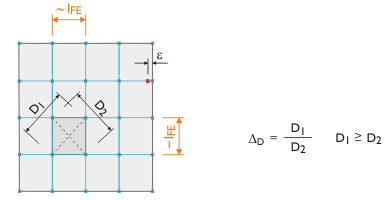Figure 7.11 Elements with diagonals D1 and D2

A curved surface is transformed into planar elements when creating the FE mesh. The inclination angle α defines the Maximum out-of-plane inclination of a single quadrangle element. This value describes the angle between the normals of two elements (see the following figure with normals between two triangular elements). If the allowable inclination angle is exceeded, it is divided into additional triangular elements.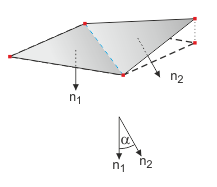Figure 7.12 Inclination angle α

If the model is defined as a plate of the type 2D - in XY, you can specify an FE mesh refinement along lines to create smaller finite elements on all lines and thus better approximate, for example, results along supported lines. The relation Δ b refers to the global mesh size. It describes the edge distance of the refinement from the lines.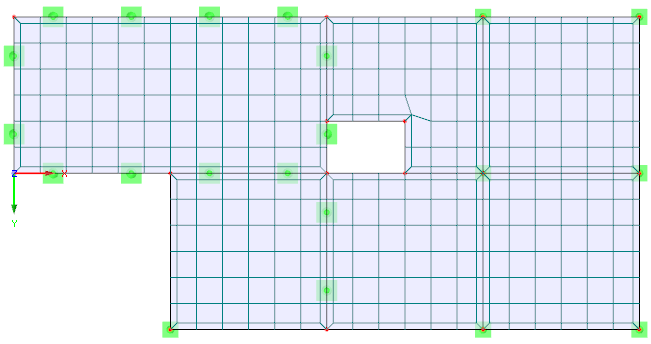Figure 7.13 FE mesh refinement along the boundary lines of a 2D slab

Select the Integrate unutilized objects into surfaces check box to also generate FE nodes on objects that do not have any specific function for a surface (e.g. free nodes without support or load, constructional lines in surfaces). This function is disabled by default so that structurally irrelevant objects do not warp the FE mesh.

Mapped mesh preferred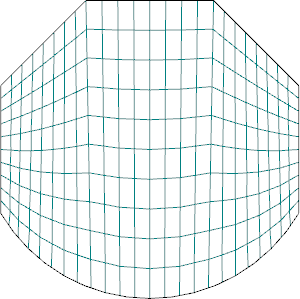Mapped mesh preferred

The Shape of finite elements can be determined with the following three options:

• Triangles and quadrangles: default setting
• Triangles only: option in case quadrangles cause strong mesh distortions
• Quadrangles only: option for higher accuracy of results

The Mapped mesh preferred option tries to align the FE mesh with the boundary lines of surfaces. This type of FE mesh generation can be individually defined for each surface (Edit Surface dialog box, FE Mesh tab).

A mapped mesh is exclusively composed of quadrangles. In general, a mapped mesh provides "more accurate" results. Since less unknown factors also occur in the equation system, this method is recommended for generating the mesh.

Solids

If nodes are very close together on a solid, you can define an automatic FE mesh refinement. Thus, the FE mesh determines the nodes correctly. The mesh size of the solid is determined from the shortest distance of the nodes. You can use the text box to limit the number of generated 3D elements.

It is possible to enter specific requirements regarding the FE mesh for each solid. This possibility is described in Chapter 4.5.